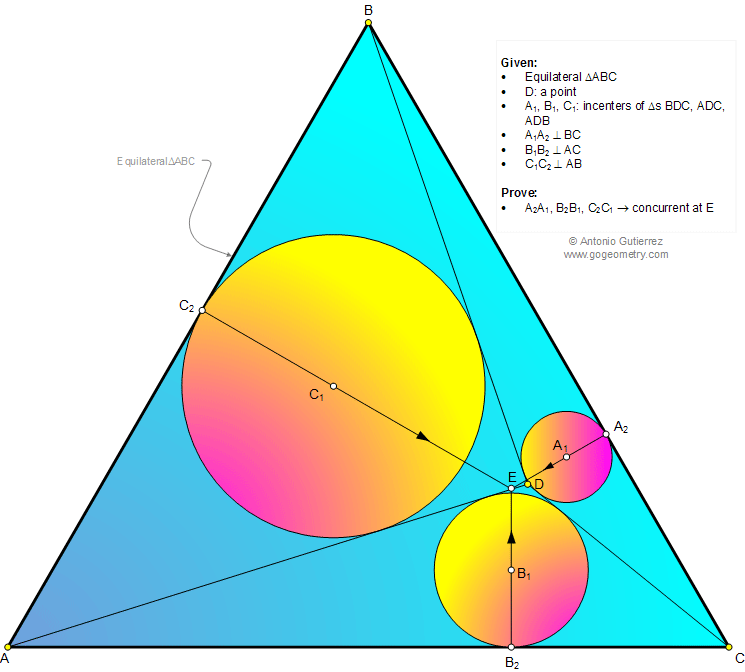< PREVIOUS PROBLEM  |  NEXT PROBLEM >

# Math Geometry Problem 1012: Equilateral Triangle, Incenters, Inscribed Circles, Perpendicular, Concurrent Lines. Level: School, College

The figure shows an equilateral triangle ABC with a point D. A1, B1, C1 are the incenters of triangles BDC, ADC, and ADB, respectively. Prove that the perpendiculars dropped from A1, B1, and C1 to BC, AC, and AB, respectively, are concurrent at a point E.Home | SearchGeometry | Problems | All Problems | Open Problems | Visual Index | 10 Problems | Problems Art GalleryArt | 1011-1020 | Triangle | Equilateral Triangle | Incenter | Circle | Perpendicular lines | Concurrent lines | Email | Solution / comment | By Antonio Gutierrez